# 20200428上证指数分析-上吊线？吊颈线？锤头线？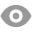640 |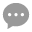6 |桥博士 | 2020-4-28 16:34:56 | 显示全部楼层 |阅读模式
 今天有一根很长出下影线：上吊线？吊颈线？锤头线？ 因为该K线形态骨骼清奇，在分时图上呈现V字型 我提前也做了一些功课，研究了一下。 一、大家今天的作业开始尝试，自己定义K线形态， A不必拘泥于一些不太重要的参数，比如 大于5倍的ATR还是大于3倍的ATR B尽量取整数，整十的最好，整五的次之 C第一重要是“破位”，即向上或向下突破，创出了新高或新低 D第二重要是K线的长度，没有破位的大K线，也有影响力的 二、代码改后以后，加载到K线图上 A上证指数20200428这一天要有买入信号 B上证指数过去20年的历史数据中，至少交易了20笔以上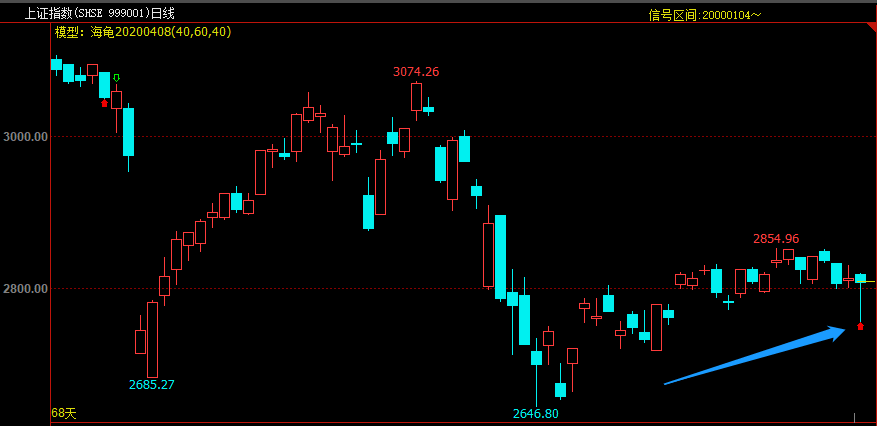下面是我写的2个参考范例，都可以达到上述要求 ///////////////////////////////////////// K线形态（长下影线）再配合一些破位的条件 //////////////////////////////////////// //定义仓位 FUND:=10000000; LOTS:=INTPART(FUND*0.4/(CLOSE+FEE));//计算手数，使每次开仓金额都是400W //定义ATR TR:=MAX(MAX((H-L),ABS(REF(C,1)-H)),ABS(REF(C,1)-L));//求最高价减去最低价，一个周期前的收盘价减去最高价的绝对值，一个周期前的收盘价减去最低价的绝对值，这三个值中的最大值 ATR:=MA(TR,26);//求N个周期内的TR的简单移动平均 //定义K线 YX:=H-L;//影线长度 ST:=ABS(O-C);//锤头K线实体 SYX:=H-MAX(O,C);//锤头K线上影线 XYX:=MIN(O,C)-L;//锤头K线下影线 //定义上证指数当日形态 SZZS1:=L<=LLV(L,10);//当日最低价破15日内的新低； SZZS2:=HHV(H,10)=HHV(H,20);//10日内的最高价创20日内的新高； SZZS3:=YX>0.5*ATR;//K线的长度大于N 倍的ATR； SZZS4:=XYX>SYX*3 AND XYX>ST*3 ;//锤头K线下影线 大于5倍的锤头K线上影线；且锤头K线下影线大于锤头K线实体长度; SZZS:=SZZS1 AND SZZS2 AND SZZS3 AND SZZS4;//上证形态：同时符合条件1、条件2、条件3、条件4; SZZS,BK(LOTS);//满足当日形态，开仓做多； BARSBK=1,SP(BKVOL);//第二天就平仓； //////////////////////////////////////////////////////////////////// 第二模型我在第一个模型基础上稍作修改，突出了海龟交易法则中开仓后，首次“创10日新低”平仓 ////////////////////////////////////////////////////////////////// //定义仓位 FUND:=10000000; LOTS:=INTPART(FUND*0.4/(CLOSE+FEE));//计算手数，使每次开仓金额都是400W //定义ATR TR:=MAX(MAX((H-L),ABS(REF(C,1)-H)),ABS(REF(C,1)-L));//求最高价减去最低价，一个周期前的收盘价减去最高价的绝对值，一个周期前的收盘价减去最低价的绝对值，这三个值中的最大值 ATR:=MA(TR,26);//求N个周期内的TR的简单移动平均 //定义K线 YX:=H-L;//影线长度 ST:=ABS(O-C);//锤头K线实体 SYX:=H-MAX(O,C);//锤头K线上影线 XYX:=MIN(O,C)-L;//锤头K线下影线 //定义上证指数当日形态 SZZS1:=L<=LLV(L,10); SZZS2:=HHV(H,10)=HHV(H,20); SZZS3:=YX>ATR; SZZS4:=COUNT(SZZS1,20)=1;//!!!!!!重点在这里 SZZS:=SZZS1 AND SZZS2 AND SZZS3 AND SZZS4; SZZS,BK(LOTS); BARSBK=1,SP(BKVOL);

 //定义仓位 FUND:=10000000; LOTS:=INTPART(FUND*0.4/(CLOSE+FEE));//计算手数，使每次开仓金额都是800W //定义ATR TR:=MAX(MAX((H-L),ABS(REF(C,1)-H)),ABS(REF(C,1)-L));//求最高价减去最低价，一个周期前的收盘价减去最高价的绝对值，一个周期前的收盘价减去最低价的绝对值，这三个值中的最大值 ATR:=MA(TR,26);//求N个周期内的TR的简单移动平均 //定义K线 YX:=H-L;//影线长度 ST:=ABS(O-C);//锤头K线实体 SYX:=H-MAX(O,C);//锤头K线上影线 XYX:=MIN(O,C)-L;//锤头K线下影线 //定义上证指数当日形态 SZZS1:=L<=LLV(L,10); SZZS2:=HHV(H,10)=HHV(H,20); SZZS3:=YX>ATR; SZZS4:=COUNT(SZZS1,20)=1;//!!!!!!重点在这里 SZZS:=SZZS1 AND SZZS2 AND SZZS3 AND SZZS4; SZZS,BK(LOTS); BARSBK=1,SP(BKVOL); 1天：胜率50%，盈亏比0.56，交易32次，胜率盈亏-0.22； 2天：胜率40.63%，盈亏比0.51，交易32次，胜率盈亏-0.39； 5天：胜率40%，盈亏比0.43，交易30次，胜率盈亏-0.43； 10天：胜率48.28%，盈亏比0.34，交易29次，胜率盈亏-0.35； 20天：胜率55.17%，盈亏比0.42，交易29次，胜率盈亏-0.22； 结论看跌。

 去掉10日最低的限制，增加今日收跌的要求： //定义仓位 FUND:=10000000; LOTS:=INTPART(FUND*0.4/(CLOSE+FEE));//计算手数，使每次开仓金额都是800W //定义ATR TR:=MAX(MAX((H-L),ABS(REF(C,1)-H)),ABS(REF(C,1)-L));//求最高价减去最低价，一个周期前的收盘价减去最高价的绝对值，一个周期前的收盘价减去最低价的绝对值，这三个值中的最大值 ATR:=MA(TR,26);//求N个周期内的TR的简单移动平均 //定义K线 YX:=H-L;//影线长度 ST:=ABS(O-C);//锤头K线实体 SYX:=H-MAX(O,C);//锤头K线上影线 XYX:=MIN(O,C)-L;//锤头K线下影线 //定义上证指数当日形态 SZZS3:=YX>0.5*ATR; SZZS4:=XYX>SYX*3 AND XYX>ST*2 ; SZZS5:=O>C;//收跌 SZZS:= SZZS3 && SZZS4 && SZZS5; SZZS,BK(LOTS); BARSBK=HOLDING_DAY,SP(BKVOL); //////////////////////////////////////////////////////////////////// 结论： 交易次数：98次 胜率：54.08% 盈利率：-5.24% 资金曲线：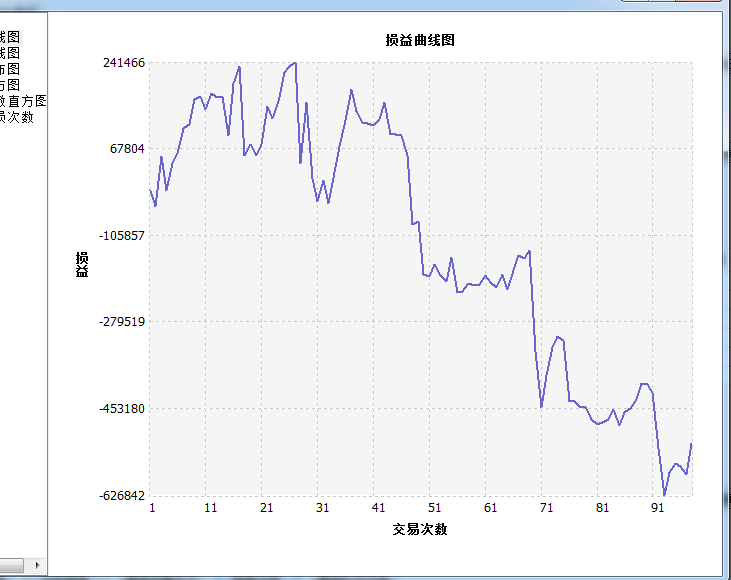从资金曲线上看，近期这种形态看跌。 结论：明天看跌。

 //定义仓位 FUND:=10000000; LOTS:=INTPART(FUND*0.4/(CLOSE+FEE));//计算手数，使每次开仓金额都是800W //定义ATR TR:=MAX(MAX((H-L),ABS(REF(C,1)-H)),ABS(REF(C,1)-L));//求最高价减去最低价，一个周期前的收盘价减去最高价的绝对值，一个周期前的收盘价减去最低价的绝对值，这三个值中的最大值 ATR:=MA(TR,26);//求N个周期内的TR的简单移动平均 //定义K线 YX:=H-L;//影线长度 ST:=ABS(O-C);//锤头K线实体 SYX:=H-MAX(O,C);//锤头K线上影线 XYX:=MIN(O,C)-L;//锤头K线下影线 //定义上证指数当日形态 SZZS1:=L<=LLV(L,10); SZZS2:=YX>1*ATR; SZZS3:=XYX>SYX*5 AND XYX>ST*3; SZZS:=SZZS1 AND SZZS2 AND SZZS3 ; SZZS,BK(LOTS); BARSBK=1,SP(BKVOL);       去掉对高点的限制条件，强化上影线对下影线和实体的系数。让交易次数尽可能靠近20.测试结果如下：       盈利率    -16.21%       胜   率      22.7%       盈亏比      0.44       交易次数    22        但是模型得分是38分，这说明是个极差的模型。这是说对盈利来说是极差，还是对预测来说是极差的？      结论看跌

 错在哪里呢？ 这是16节课里老师的第一个改变定型的代码： //定义仓位 FUND:=10000000; LOTS:=INTPART(FUND*0.4/(CLOSE+FEE));//计算手数，使每次开仓金额都是400W //定义ATR TR:=MAX(MAX((H-L),ABS(REF(C,1)-H)),ABS(REF(C,1)-L));//求最高价减去最低价，一个周期前的收盘价减去最高价的绝对值，一个周期前的收盘价减去最低价的绝对值，这三个值中的最大值 ATR:=MA(TR,26);//求N个周期内的TR的简单移动平均 //定义K线 KX:=H-L;//K线长度 ST:=ABS(O-C);//锤头K线实体 SYX:=H-MAX(O,C);//锤头K线上影线 XYX:=MIN(O,C)-L;//锤头K线下影线 //定义上证指数当日形态 SZZS1:=L<=LLV(L,10);//当日最低价破10日内的新低； SZZS2:=HHV(H,10)=HHV(H,20);//10日内的最高价创20日内的新高； SZZS3:=KX>0.5*ATR;//K线的长度大于N 倍的ATR； SZZS4:=XYX>(SYX+ST)*1.5 ;//锤头K线下影线 大于5倍的锤头K线上影线；且锤头K线下影线大于锤头K线实体长度; SZZS:=SZZS1 AND SZZS2 AND SZZS3 AND SZZS4;//上证形态：同时符合条件1、条件2、条件3、条件4; SZZS,BK(LOTS);//满足当日形态，开仓做多； BARSBK=1,SP(BKVOL);//第二天就平仓； 结果是：交易信号54个，次数27次（老师上课引用的数据是到2020-4-28止的，我引用的数据是2020-4-30止的） 我的想法，就是的把锤头线的实体部分，分出阳锤头和阴锤头来，就给K线定义部分作了修改，其余代码未改变。修改部分的代码如下： //定义K线 KX:=H-L;//K线长度 ST:=C-O AND C>O;//锤头K线实体 SYX:=H-C;//锤头K线上影线 XYX:=O-L;//锤头K线下影线 测试结果，交易次数变多了，信号变为212次，交易次数为106次并且2020-4-28和2020-3-17这两天明明锤头是阴锤头，也有信号了。请老师和同学帮忙看看，我修改的代码是哪里出错了？谢谢！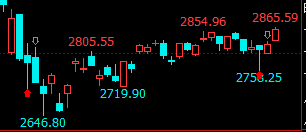ST:=C-O AND C>O;//锤头K线实体 这句话改错了， 之前来定义的是实体的长度

 测试 ------2021年5.6日k线 //定义仓位 FUND:=10000000; LOTS:=INTPART(FUND*0.4/(CLOSE+FEE));//计算手数，使每次开仓金额都是400W //定义ATR TR:=MAX(MAX((H-L),ABS(REF(C,1)-H)),ABS(REF(C,1)-L));//求最高价减去最低价，一个周期前的收盘价减去最高价的绝对值，一个周期前的收盘价减去最低价的绝对值，这三个值中的最大值 ATR:=MA(TR,26);//求N个周期内的TR的简单移动平均 //定义K线 YX:=H-L;//影线长度 ST:=ABS(O-C);//锤头K线实体 SYX:=H-MAX(O,C);//锤头K线上影线 XYX:=MIN(O,C)-L;//锤头K线下影线 //定义上证指数当日形态 SZZS1:=YX>ATR;//K线的长度大于N 倍的ATR； SZZS2:=XYXSYX  AND XYX>ST;//锤头K线下影线 小于于锤头K线上影线；2倍锤头K线下影线 大于于锤头K线上影线；且锤头K线下影线大于锤头K线实体长度; SZZS3:=O>C; SZZS:=SZZS1 AND SZZS2 AND SZZS3;//上证形态：同时符合条件1、条件2、条件3 SZZS,BK(LOTS);//满足当日形态，开仓做多； BARSBK=1,SP(BKVOL);//第二天就平仓；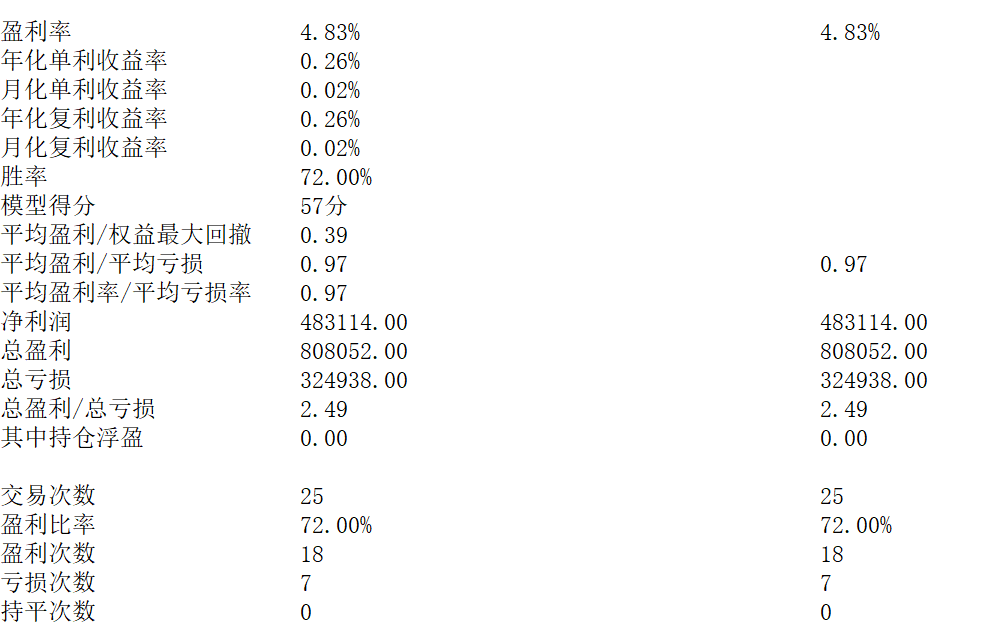您需要登录后才可以回帖 登录 | 立即注册 本版积分规则 回帖后跳转到最后一页177主题 2273积分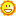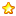2273